Our World Statistics Day conversations have been a great reminder of how much statistics can inform our lives. Do you have an example of how statistics has made a difference in your life? Share your story with the Community!
Choose Language Hide Translation Bar
Highlighted

## Is there a way to write a script to that graphs 2 different types of plots in one graph?

Hi, I am fairly new to jmp and am trying to write a script to automate something I have been able to do in other software. I am not sure what I am wanting to do is possible. I am trying to use some data to overlay 2 graphs, one is a scatter plot and the other is a line graph. I am able to do the scatter plot, but cannot figure out how to include the line graph one as well.

I have included some images of the 2 graphs I want to overlay, and also an example with other software of how it should look. I also will include what I have so far for my script.

Thanks in advance for any assistance.

``````framex_value = "";
w = New Window( "Frame size X", // opens a window with a title and this content...
<<Return Result,
Border Box( top( 20 ), bottom( 20 ), Left( 20 ), Right( 20 ), // window dressing
V List Box( // V and H lists nest to organize the display boxes
H Center Box( Text Box( "Frame size X" ) ), // a second title, centered
Spacer Box( size( 1, 30 ) ), // a little vertical space
H List Box( Text Box( "Frame size X: " ), framex = Number Edit Box( "" ) ), // data entry
Spacer Box( size( 1, 10 ) ), // a little vertical space
H Center Box( // center the button
Button Box( "Create graph", // this script runs when the button is pressed...
framex_value = framex << get text();
values1 = {framex_value, framex_value};
values2 = {1, 0.92, 0.92, 1};

column("X coordinate")<<data type(Numeric)<<Modeling Type(Continuous)<<Format(Best,12);
New Column ( "New Y", Numeric, Nominal, Width( 5), Formula(Round(Y coordinate)));
dt =Current Data Table();
dt << select where( :New Y != 50503 );
dt << delete rows;
New Column ( "Normalized", Numeric, Continuous, Width( 10 ), Precision ( 7 ), Formula(IntenCD/Col Max(IntenCD)) );

New Column ( "Frame X", Numeric, Continuous, Width( 10 ), Precision ( 7 ), set values( values1 ));
New Column ( "Frame X 2", Numeric, Continuous, Width( 10 ), Precision ( 7 ), Formula(76200 - (Frame X/2)));
New Column ( "Frame Y", Numeric, Continuous, Width( 10 ), Precision ( 7 ), set values( values2 ));

Graph Builder(
Variables( X( :X coordinate ), Y( :Normalized ) ),
Elements( Points( X, Y, Legend( 9 ) ) ),

//Variables( X( :Frame X ), Y( :Frame Y ) ),
Elements( Line( X, Y, Legend( 5 ), Row order( 1 ) ) ),

SendToReport(
Dispatch(
{},
"X coordinate",
ScaleBox,
{Min( 10000 ), Max( 140000 ), Inc( 10000 ), Minor Ticks( 1 )}
),
Dispatch(
{},
"Normalized",
ScaleBox,
{Min( 0.91 ), Max( 1.01 ), Inc( 0.002 ), Minor Ticks( 1 )}
)
)
);

// launch a platform to analyze the data...
//dt << Distribution( Continuous Distribution( Column( :a ), Quantiles( 0 ), Summary Statistics( 0 ), Vertical( 0 ), Outlier Box Plot( 0 ) ) );
// optionally, close the dialog. Or, you might want to run it again...
w << closeWindow; // just the dialog, not the report
)
)
)
)
);``````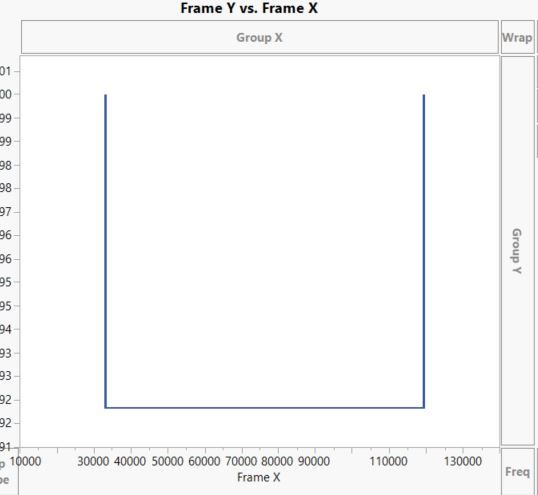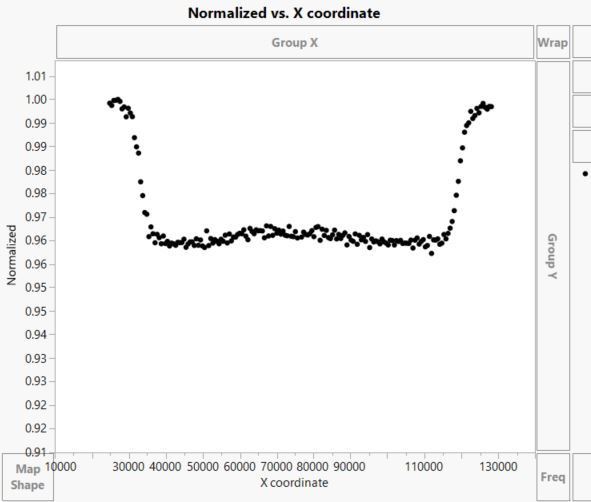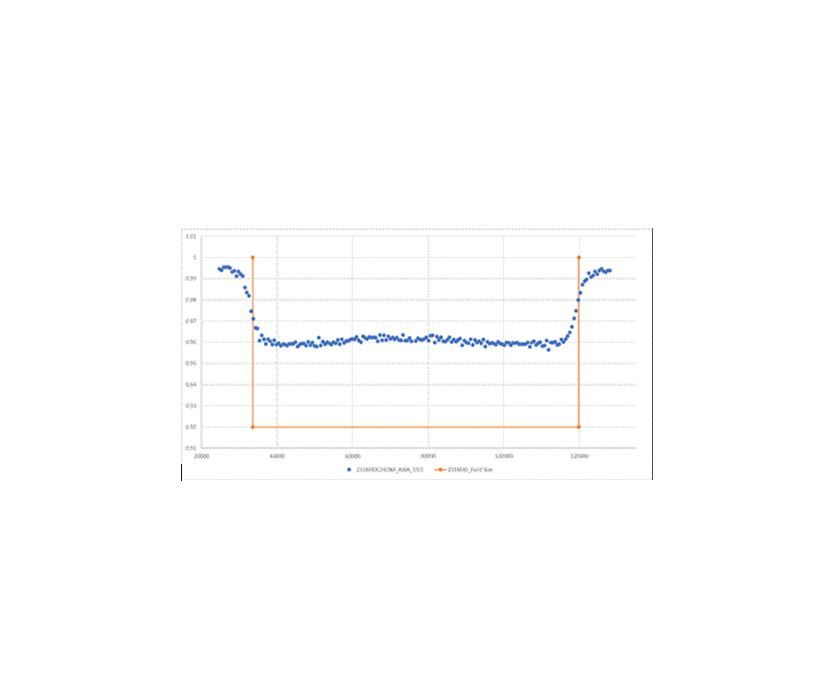3 ACCEPTED SOLUTIONS

Accepted Solutions
Highlighted

## Re: Is there a way to write a script to that graphs 2 different types of plots in one graph?

Here are 2 solutions.

First solution creates the graph with just the X Coordinate and the Normalized data, and then using Add Graphics Script, adds in the line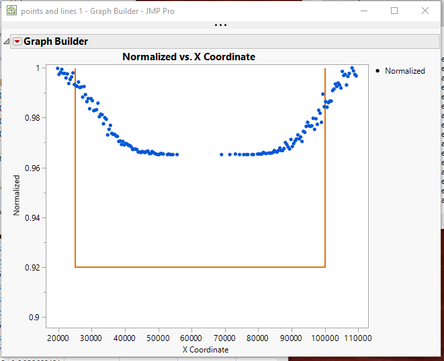The second solution, adds in extra rows to the data table, and 1 extra column, and then by specifying which variables to show points for, and which one to show a line for, the Graph Builder comes up with the final plot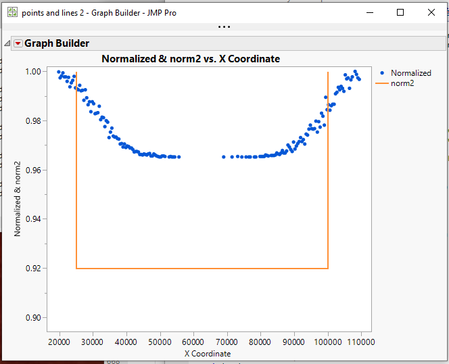The 2 data tables are attached and the scripts for each version are saved to the respective data tables.

Jim
Highlighted

## Re: Is there a way to write a script to that graphs 2 different types of plots in one graph?

2. Here is an example
``````Names default to here(1);
framex_value = 5000;

xMat = matrix(76200 - framex_value) |/ matrix(76200 - framex_value) |/
matrix(76200 + framex_value) |/ matrix(76200 + framex_value);``````
Jim
Highlighted

## Re: Is there a way to write a script to that graphs 2 different types of plots in one graph?

You are changing the value of "framex_value" from numeric to character when you specify

`` framex_value = framex << get text();``

You are telling JMP to get the value of framex as "text"

You should be specifying

`` framex_value = framex << get ;``

Look in the Scripting Guide under "NumberEditBox" and you will see all messages that can be handled by "NumberEditBox", and examples for each one.

On another related issue you are specifying

``framex = Number Edit Box( "" )``

which is telling JMP to apply a character value to you Numeric Input Box.  Because it is a numeric field, you should be specifying either a valid number, or a period, "."   The "." is handled as a missing value.

Jim
8 REPLIES 8
Highlighted

## Re: Is there a way to write a script to that graphs 2 different types of plots in one graph?

Here are 2 solutions.

First solution creates the graph with just the X Coordinate and the Normalized data, and then using Add Graphics Script, adds in the lineThe second solution, adds in extra rows to the data table, and 1 extra column, and then by specifying which variables to show points for, and which one to show a line for, the Graph Builder comes up with the final plotThe 2 data tables are attached and the scripts for each version are saved to the respective data tables.

Jim
Highlighted

## Re: Is there a way to write a script to that graphs 2 different types of plots in one graph?

This is perfect, thanks so much. I appreciate it so much. I have been trying to figure out how to do this for quite a while.....I was able to get it to work on my dataset....now I just need to see about adding the values for the line graph into the columns. Using your column names, the X coordinates for the line will be dependent on a user input value, so they will change, but the norm2 will always be the same 4 values. I see you replied to the other post I made about this. I will review that one. Thanks again.

Highlighted

## Re: Is there a way to write a script to that graphs 2 different types of plots in one graph?

I am responding back, to urge you to reevaluate the first option that I showed you.  It has the distinct advantage of not messing with your data.  Below is a rework of the script that Graph Builder produced for the first option.  The reworked code will show you how to incorporate calculated values directly into the graphical output.

``````Names Default To Here( 1 );
dt = Current Data Table();

// Here it is just being assigned, but you can easily create it in your code
xMat = [25000, 25000, 100000, 100000];
// The yMat is static, so it would be input as is
yMat = [1, 0.92, 0.92, 1];

gb = dt << Graph Builder(
Size( 534, 456 ),
Show Control Panel( 0 ),
Variables( X( :X Coordinate ), Y( :Normalized ) ),
Elements( Points( X, Y, Legend( 5 ) ) )
);

Report( gb )[FrameBox( 1 )] << Add Graphics Script(
Pen Color( "orange" );
Pen Size( 2 );
Line( xmat, ymat );
);
report(gb)[AxisBox(2)] << min(.91);``````
Jim
Highlighted

## Re: Is there a way to write a script to that graphs 2 different types of plots in one graph?

@txnelson

This is great, I really like this solution. Thanks for sending this one.

Could I bother you with one more question? I am struggling with getting the calculated values into the xMat. My program asks the user for a value, I take that value and the calculation for the first two xMat values should be calculated (76200-"user input value"), and the second two xMat values would be calculated (76200 + "user input value"). Thanks in advance for any assistance.

Highlighted

## Re: Is there a way to write a script to that graphs 2 different types of plots in one graph?

2. Here is an example
``````Names default to here(1);
framex_value = 5000;

xMat = matrix(76200 - framex_value) |/ matrix(76200 - framex_value) |/
matrix(76200 + framex_value) |/ matrix(76200 + framex_value);``````
Jim
Highlighted

## Re: Is there a way to write a script to that graphs 2 different types of plots in one graph?

Thanks for this example. I don't know if I am bringing in the user input incorrectly or not but I get an error when I add this to the script and try to run it.

Cannot convert argument to a number [or matrix] in access or evaluation of 'Subtract' , 76200 - /*###*/framex_value/*###*/.

I have attached my script again with all the updates if needed to help.

Thanks again in advance. I really appreciate the help.

``````framex_value = "";
w = New Window( "Frame size X", // opens a window with a title and this content...
<<Return Result,
Border Box( top( 20 ), bottom( 20 ), Left( 20 ), Right( 20 ), // window dressing
V List Box( // V and H lists nest to organize the display boxes
H Center Box( Text Box( "Frame size X" ) ), // a second title, centered
Spacer Box( size( 1, 30 ) ), // a little vertical space
H List Box( Text Box( "Frame size X: " ), framex = Number Edit Box( "" ) ), // data entry
Spacer Box( size( 1, 10 ) ), // a little vertical space
H Center Box( // center the button
Button Box( "Create graph", // this script runs when the button is pressed...
framex_value = framex << get text();

column("X coordinate")<<data type(Numeric)<<Modeling Type(Continuous)<<Format(Best,12);
New Column ( "New Y", Numeric, Nominal, Width( 5), Formula(Round(Y coordinate)));
dt =Current Data Table();
dt << select where( :New Y != 50503 );
dt << delete rows;
New Column ( "Normalized", Numeric, Continuous, Width( 10 ), Precision ( 7 ), Formula(IntenCD/Col Max(IntenCD)) );

// Here it is just being assigned, but you can easily create it in your code
xMat = matrix(76200 - framex_value) |/ matrix(76200 - framex_value) |/
matrix(76200 + framex_value) |/ matrix(76200 + framex_value);
// The yMat is static, so it would be input as is
yMat = [1, 0.92, 0.92, 1];

gb = dt << Graph Builder(
Size( 534, 456 ),
Show Control Panel( 0 ),
Variables( X( :X Coordinate ), Y( :Normalized ) ),
Elements( Points( X, Y, Legend( 5 ) ) )
);

Report( gb )[FrameBox( 1 )] << Add Graphics Script(
Pen Color( "orange" );
Pen Size( 2 );
Line( xmat, ymat );
);
report(gb)[AxisBox(2)] << min(.91);

w << closeWindow; // just the dialog, not the report
)
)
)
)
);``````

Highlighted

## Re: Is there a way to write a script to that graphs 2 different types of plots in one graph?

You are changing the value of "framex_value" from numeric to character when you specify

`` framex_value = framex << get text();``

You are telling JMP to get the value of framex as "text"

You should be specifying

`` framex_value = framex << get ;``

Look in the Scripting Guide under "NumberEditBox" and you will see all messages that can be handled by "NumberEditBox", and examples for each one.

On another related issue you are specifying

``framex = Number Edit Box( "" )``

which is telling JMP to apply a character value to you Numeric Input Box.  Because it is a numeric field, you should be specifying either a valid number, or a period, "."   The "." is handled as a missing value.

Jim
Highlighted

## Re: Is there a way to write a script to that graphs 2 different types of plots in one graph?

Perfect, my script is working awesome! Thanks again for all your help!

Article Labels